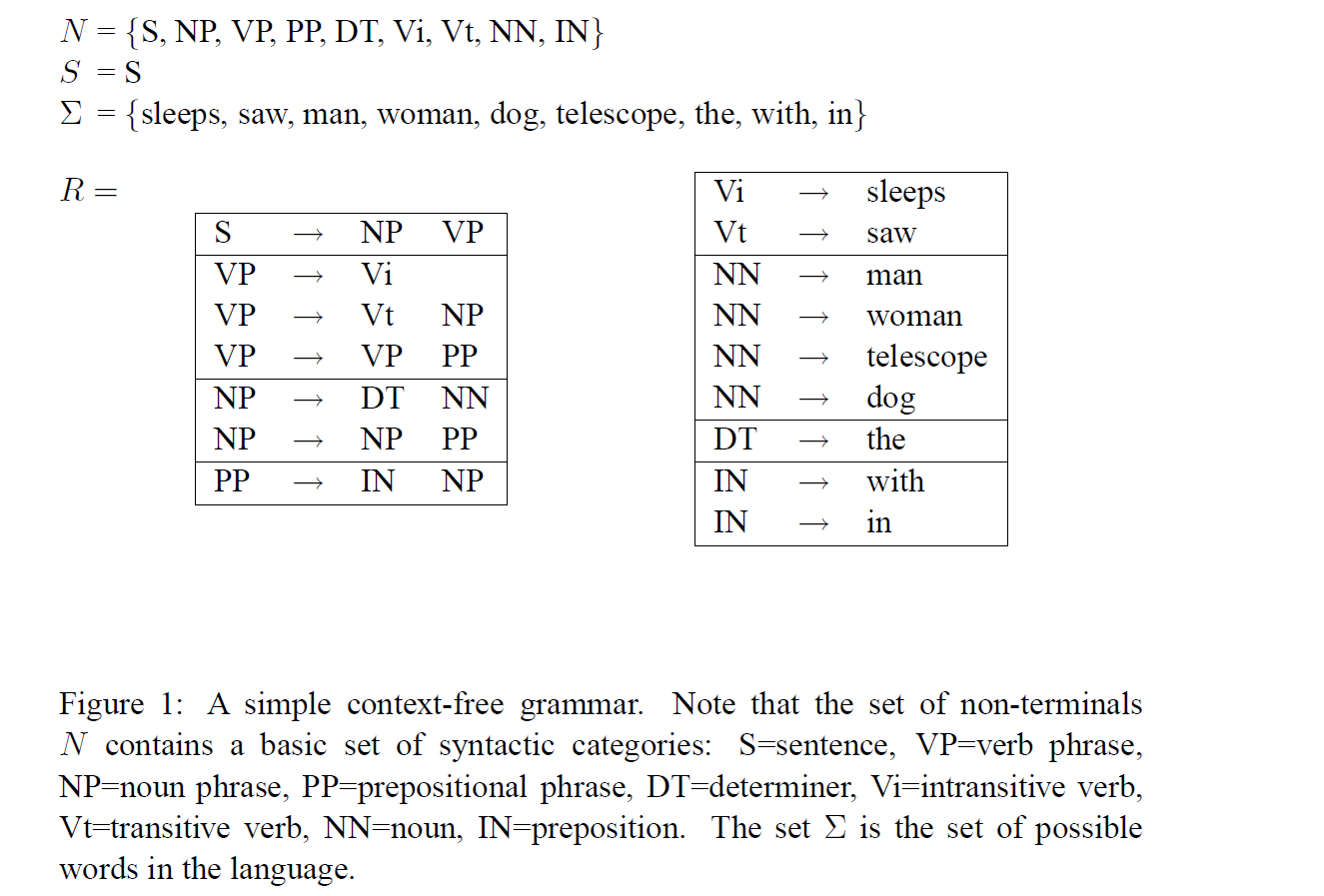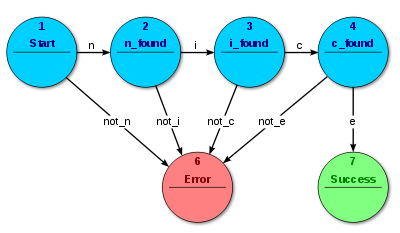## 句法模式识别(1)-句法模式识别介绍 2015.11.20

##字母表##

 字母表$V$是一个有穷的符号集，句子$x$ 是由$V$中的符号形成的有穷长度的串, $x$的长度记作 ，表示$\left x \right$表达式中符号的数目。空串记作 $\lambda$，是一个不包含任何符号的串，它的长度为0 。字母表在我们的日常生活中很常见比如说在英语中字母表就代表26个英文字母。

${V^+}={V^*}-\left\{\lambda \right\}$

$\begin{array}{l} {V^*} = \left\{ {\lambda ,a,c,aa,ac, \cdots } \right\}\ {V^ + } = \left\{ {a,c,aa,ac, \cdots } \right\} \end{array}$

##形式语言##

$G = (N,\sum ,R,S)$##自动机##